# Texas Go Math Grade 5 Lesson 10.3 Answer Key Number Patterns in Graphs

Refer to our Texas Go Math Grade 5 Answer Key Pdf to score good marks in the exams. Test yourself by practicing the problems from Texas Go Math Grade 5 Lesson 10.3 Answer Key Number Patterns in Graphs.

## Texas Go Math Grade 5 Lesson 10.3 Answer Key Number Patterns in Graphs

Unlock the Problem

Sona has some dimes. Adil offers to give her nickels for her dimes.
The graph shows the relationship between the number of dimes Sona gives to
Adil and the number of nickels she receives in exchange. Find a rule to describe the pattern.STEP 1: Write number pairs that relate the number of dimes to the number of nickels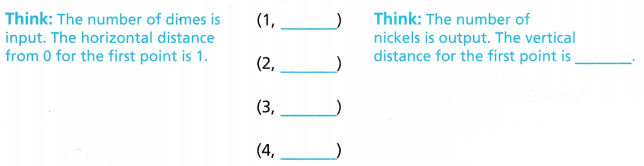STEP 2 Describe the relationship between the number of dimes and the number of nickels.
The output is __________ times the input. The pattern uses multiplication.

STEP 3 Write the rule. Let n stand for number of nickels, and d stand for the number of dimes.
n = __________ × ___________
Think: The relationship is multiplicative. Use multiplication to write the rule.

Step 1:
Pairs that relate between Sona and Adil the number of dimes to the
number of nickels are (1,2), (2,4), (3,6), (4,8),
Step 2:
The output is 2 times the input,
Step 3:
Rule: n = d X 2,

Explanation:
Step 1:
As the number of dimes is the input, the horizontal distance from
0 for the first point is 1 and the number of nickels is output the vertical distance
for the first point is 2, So the pairs that relate between Sona and Adil
the number of dimes to the number of nickels are (1,2), (2,4), (3,6), (4,8),
Step 2:
By seeing the pairs the relationship between the number of dimes is
twice the number of nickels. so the output is two times the input.
The pattern uses multiplication,
Step 3:
Let n stand for number of nickels, and d stand for the number of dimes.
as the relationship is multiplicative. Using multiplication to write the rule as
n = d X 2.

Math Idea

An additive pattern uses addition ¡n its rule. A multiplicative pattern uses multiplication in its rule.

Example

Find the rule for the pattern shown in the graph.

Jack mails some magazines in a box. The graph shows the relationship between
the number of magazines and the total weight of the magazines and the box.STEP 1: Write number pairs that relate the number of magazines and the total weight.
(1, ______)
(2, ______)
(3, ______)
(4, ______)

STEP 2: Describe the relationship between the number of magazines and the weight.
The output is the sum of the number of magazines and __________.

STEP 3: Write the rule. Let w stand for total weight, and m stand for the number of magazines.
w = __________ + __________
Pairs are (1,3), (2,4), (3,5), (4,6),
The output total weight is the sum of the number of magazines and 2,
rule is w = m + 2,

Explanation:
Step 1: Number pairs that relate the number of magazines and the total weight is
(1,3), (2,4), (3,5), (4,6),
Step 2: By seeing the graph The output is the sum of the number of magazines and 2
the relationship between the number of magazines and the weight.
The output is the sum of the number of magazines and 2 by using
addition pattern and let w stand for total weight, and
m stand for the number of magazines the rule is  w = m + 2.

Math Talk
Mathematical Processes

Explain what the 2 stands for in w = m + 2.
2 stands for adding 2 more plus number of magazines we get the weight,

Explanation:
As w = m + 2 here 2 stands we are adding 2 more to the
number of magazines we will get the weight.

Share and Show

Is the pattern additive or multiplicative? Find the rule for the pattern.

Question 1.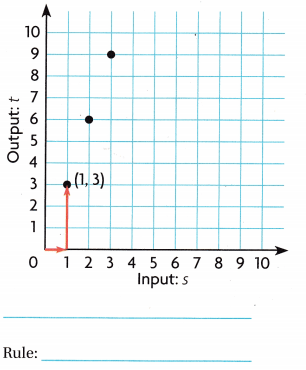Pattern is multiplicative,
Rule : t = s X 3,

Explanation:
Step 1: Number pairs that relate input s and output t we see
(1,3),(2,6), (3,6),
Step 2:  By seeing the graph the relationship between the input and output is
the output t is multiplication of 3 times with input s by using
multiplication pattern rule is  t = s X 3.

Question 2.Rule : t = s + 3,

Explanation:
Step 1: Number pairs that relate input s and output t we see
(1,4),(2,5), (3,6),
Step 2:  By seeing the graph the relationship between the input and output is
the output t is addition of 3 more with input s by using
addition pattern rule is  t = s + 3.

Problem Solving

Question 3.
H.O.T. Write Math Explain the differences between the graphs in Exercises 1 and 2.
One is multiplicative pattern and other is additive pattern,

Explanation:
The main difference between the graphs in Exercise 1 and 2 is
the first 1 is multiplicative pattern with steeper(larger) slope and linear and
seeing relations between input and output,
In the second graph it is additive pattern with linear and deeper(closer) slope
and seeing relations between input and output.

Question 4.
Write Math Explain how you can tell whether a pattern shown in a graph is additive or multiplicative.
By seeing the graph and points
origin, slope is deeper or steeper, linear
and relations between input and outputs,

Explanation:
In additive graph it will not start from origin and slope
will be deeper(closer) and it will be linear and we see the
relationship between input and output,
In multiplicative graph it can will start from origin and slope
will be steeper(increases) and it will be linear and we see the
relationship between input and output.

Problem Solving

Use the graph for 5-6.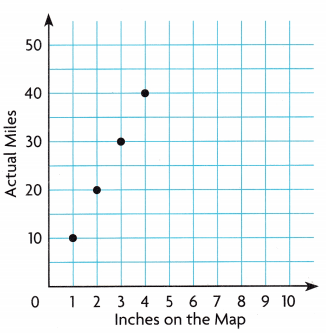Question 5.
H.O.T. Multi-Step Maryanne’s map uses a scale to show how many miles each inch on the map represents.
Use the pattern shown in the graph to find the rule for calculating the actual distance.
Use in for number of miles and j for number of inches.Rule in = j X 10,

Explanation:
Given Maryanne’s map uses a scale to show how many miles each inch on the map represents.
Step 1: Number pairs that relate input  and output  is
(1,0),(2,20), (3,30),(4,40),
Step 2:  By seeing the graph the relationship between
in for number of miles and j for number of inches on map rule is
the number of miles is multiplication of 10 times with number of inches on the map
using multiplication pattern rule is (actual distance) in  = j X 10
Used the pattern shown in the graph to find the rule for calculating the actual distance.

Question 6.
H.O.T. If the distance on the map is 2.5 inches, how many miles is the actual distance?
Explain how you calculated the actual distance.
The actual distance is 25 miles,

Explanation:
Given the distance on the map is 2.5inches to calculate
the actual distance by using the graph and rule of question 5 we have
(actual distance) in  = j X 10(where j is number of inches on the map)
substituting j = 2.5 we get in = 2.5 X 10 = 25 miles the actual distance.

Question 7.
H.O.T. Apply Olivia uses 1 red button for the nose and 2 blue buttons
for the eyes for each rag doll she makes.
Would the pattern comparing the number of nose buttons to
the total number of buttons be multiplicative or additive? Explain.
The number of nose buttons to total number of buttons
will be multiplicative,

Explanation:
Given Olivia uses 1 red button for the nose and 2 blue buttons
for the eyes for each rag doll she makes. If n is the total number of buttons
let r is red button for the nose and b for blue button we have
1 rag doll = 3 buttons = 1 red button + 2 blue buttons
means the total number of buttons is
depending upon number of red buttons and also on
blue buttons which means it is multiplicative
if 2 rag dolls = 3 x 2 = 6 buttons = (2 X 1r + 2 X 2b) = 2 + 4 = 6.

Fill in the bubble completely to show your answer. Use the graph.

Question 8.
The graph shows the number of pounds of fish the seals at an aquarium eat each day.
Which statement and equation about the relationship is true?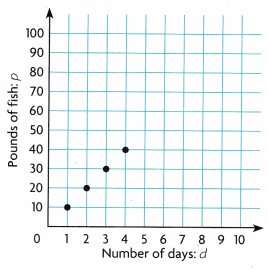(A) additive pattern, d = p + 3
(B) addition pattern, p = d + 3
(C) multiplicative pattern, p = 10 × d
(D) multiplicative pattern, d = 3 × p
(C) multiplicative pattern, p = 10 × d is true,

Explanation:
Given the graph shows the number of pounds of fish the seals
at an aquarium eat each day the pattern is if
p is the number of pounds of fish the seals and d is number of days
Step 1: Number pairs that relate input d and output p we see
(1,10),(2,20), (3,30),(4,40),
Step 2:  By seeing the graph the relationship between the input and output is
multiplicative of 10 times with input d is output p by using
multiplicative pattern rule is  p = 10 X d which exactly matches with (C).

Use the graph for 9-10.Question 9.
The graph shows the relationship between the number of days and
the amount of food that a bottlenose dolphin eats.
Which point on the graph shows the amount of food that the dolphin consumes in two days?
(A) point A
(B) point B
(C) point C
(D) point D
(C) point C,

Explanation:
Given the graph shows the relationship between the number of days and
the amount of food that a bottlenose dolphin eats. If we see points on the
graph Point A is day 0, Point B is day 1, Point C is day 2 and Point D is day 3,
So point on the graph that shows the amount of food that the
dolphin consumes in two days is Point C matches with (C).

Question 10.
Multi-Step If  s is the amount of food a bottlenose dolphin cats in a
day and n is the number of days, which of the following describes the
rule for the pattern shown in the graph?
(A) s = n + 23
(B) s = n × 23
(C) n = s × 23
(D) n = s + 23
(A) s = n + 23,

Explanation:
If  s is the amount of food a bottlenose dolphin cats in a
day and n is the number of days, Number pairs that relate
input n and output s we see
(1,23),(2,46), (3,69), the following describes the
rule for the pattern shown in the graph the relationship between the
input number of days n and output s is the amount of food a
bottlenose dolphin cats in a day is additive of 23 more with input n
is output s by using additive pattern rule is  s = n + 23
which exactly matches with (A).

Texas Test Prep

Question 11.
Which of the following rules describes the pattern in the graph?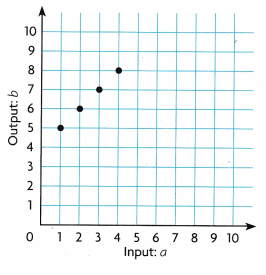(A) a = 4b
(B) b = 4a
(C) b = 4 + a
(D) a = 4 + b
(C) b = 4 + a,

Explanation:
Number pairs that relate input a and output b we see
(1,5), (2,6), (3,7), (4, 8) the following describes the
rule for the pattern shown in the graph the relationship between the
input a and output b is additive of 4 more with input a is output b by using
additive pattern rule we have b = 4 + a which exactly matches with (C).

### Texas Go Math Grade 5 Lesson 10.3 Homework and Practice Answer Key

Is the pattern additive or multiplicative? Write the rule for the pattern.

Question 1.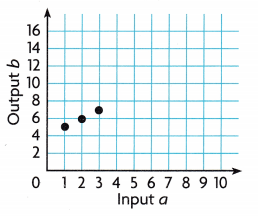Rule is b = a + 4,

Explanation:
Number pairs that relate input a and output b we see
(1,5), (2,6), (3,7) the following describes the
rule for the pattern shown in the graph the relationship between the
input a and output b is additive of 4 more with input a is output b by using
additive pattern rule is b = a + 4.

Question 2.Pattern is Multiplicative,
Rule is d = c X  4,

Explanation:
Number pairs that relate input c and output d we see
(1,4), (2,8), (3,12) the following describes the
rule for the pattern shown in the graph the relationship between the
input c and output d is multiplicative of 4 more with input c is output d
by using multiplicative pattern rule is d = c X 4.

Question 3.Pattern is Multiplicative,
Rule is f = e X  5,

Explanation:
Number pairs that relate input e and output f we see
(1,5), (2,10), (3,15) the following describes the
rule for the pattern shown in the graph the relationship between the
input e and output f is multiplicative of 5 more with input e is output f
by using multiplicative pattern rule is f = e X 5.

Question 4.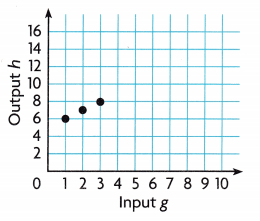Rule is h = g + 5,

Explanation:
Number pairs that relate input a and output b we see
(1,6), (2,7), (3,8) the following describes the
rule for the pattern shown in the graph the relationship between the
input g and output h is additive of 5 more with input g  is output h
by using additive pattern rule is h = g + 5.

Problem SolvingQuestion 5.
Patrice rents a rowboat for several hours. Use the pattern shown
in the graph to write the rule for calculating the cost of renting the rowboat.
Use c for the cost and h for the number of hours.
The rule for calculating the cost of renting the rowboat is
c = h X $15, where c for the cost and h for the number of hours, Explanation: Given Patrice rents a rowboat for several hours the pattern shown in the graph if c is for the cost and h for the number of hours is Number pairs that relate hours h and cost c we see (1,15), (2,30), (3,45) the following describes the rule for the pattern shown in the graph the relationship between the hours h and costs c is multiplicative of 15 times by using multiplicative pattern rule is c = h X$15.

Question 6.
If Patrice rents the boat for 4 hours, what is the cost of the rental?
The cost of the rental is $60, Explanation: we have the rule for calculating the cost of renting the rowboat is c = h X$15, where c for the cost and h for the number of hours,
so if Patrice rents the boat for 4 hours,  the cost of the rental is
c= 4 X $15 =$60.

Lesson Check

Question 7.
The graph shows the relationship between the number of hours
Ivana bikes and the distance she travels. How many miles does Ivana bike in 4 hours?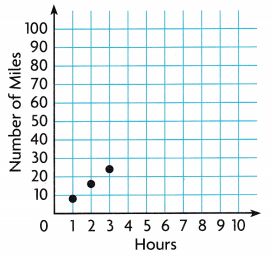(A) 28 miles
(B) 12 miles
(C) 32 miles
(D) 30 miles
(C) 32 miles,

Explanation:
Given the graph shows the relationship between the number of hours
Ivana bikes and the distance she travels
Step 1: Number pairs that relate hours h and number of miles m we see
(1,8),(2,16), (3,24),
Step 2:  By seeing the graph the relationship between the input and output is
multiplicative of 8 times with input hours h is output number of miles m by using
multiplicative pattern rule is  m = 8 X h so number of miles does
Ivana bike in 4 hours is 8 X 4 = 32 miles which matches with (C).

Question 8.
Rashid uses cubes to build towers. The graph shows the relationship between
the number of towers, t, and the number of cubes, c, he uses.
Which equation describes this relationship?(A) c = 12 + t
(B) c = 12t
(C) t = 12 + c
(D) t = 12c
(B) c = 12t,

Explanation:
Given Rashid uses cubes to build towers.
The graph shows the relationship between
the number of towers t and the number of cubes c,
Step 1: Number pairs that relate the number of towers t and the number of cubes  c,
we see (1,12),(2,24), (3,36),
Step 2:  By seeing the graph the relationship between the input and output is
multiplicative of 12 times with input the number of towers t  is
output  by using the number of cubes c multiplicative pattern rule is
c = 12 X t therefore equation describes this relationship is (B).

Question 9.
Multi-Step For every $1 Paula donates to her favorite charity, her mom donates$3. Which of the following identifies the pattern and
the amount her mom donates if Paula donates $6?(A)$18, multiplicative
(B) $24, multiplicative (C)$24, additive
(D) $12, additive Answer: (A)$18, multiplicative,

Explanation:
Given for every $1 Paula donates to her favorite charity, her mom donates$3, The graph shows the relationship between
Paula donation as p and mom donation as m
Step 1: Number pairs that relate the Paula donation as p and mom donation m
we see (1,3),(2,6), (3,9),
Step 2:  By seeing the graph the relationship between the input and output is
multiplicative of 3 times with input Paula donation p  is
output  mom donation as m by using multiplicative pattern rule is
m = 3 X p therefore the amount her mom donates if Paula donates $6 is 3 X$6 = $18 matches with (A)$18, multiplicative.

Question 10.
Multi-Step The graph shows how much time Christina spends making
flower arrangements to sell. How many baskets can she make in two hours?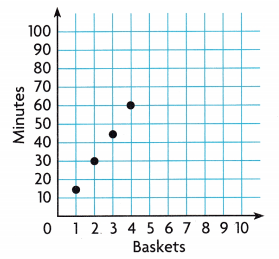(A) 15
(B) 4
(C) 30
(D) 8
(D) 8

Explanation:
Given the graph shows how much time Christina spends making
flower arrangements to sell. Number of baskets can she make in two hours
as we know 2 hours means 2 X 60 minutes = 120 minutes,
Step 1: Number pairs that relate the Christina as b means baskets and
minutes as m we see as (1,15),(2,30), (3,45),(4,60),
Step 2:  By seeing the graph the relationship between the input and output is
multiplicative of 15 times with input baskets b  is
output  minutes as m by using multiplicative pattern rule is
m = b X 15, so for 120 minutes means 120/15 = 8 baskets,
which matches with (D).

Scroll to Top